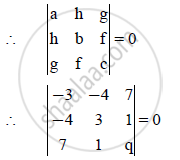HSC Arts 12th Board ExamMaharashtra State Board
Share

# Find the Values of P and Q, If the Following Equation Represents a Pair of Perpendicular Lines: Px2 − 8xy + 3y2 + 14x + 2y + Q = 0. - HSC Arts 12th Board Exam - Mathematics and Statistics

ConceptPair of Straight Lines Condition for Perpendicular Lines

#### Question

Find the values of p and q, if the following equation represents a pair of perpendicular lines:
px2 − 8xy + 3y2 + 14x + 2y + q = 0.

#### Solution

Given equation is px2 − 8xy + 3y2 + 14x + 2y + q = 0

Comparing with

ax2 + 2hxy + by2 + 2gx + 2fy + c = 0, we get

a = p, h = − 4, b = 3, g = 7, f = 1, c = q.

The given equation represents a pair of lines perpendicular to each other

∴ a + b = 0

∴ p + 3 = 0

∴ p = −3

Also, the given equation represents a pair of lines∴ −3(3q − 1) + 4( − 4q − 7) + 7(− 4 − 21) = 0

∴ −9q + 3 − 16q − 28 − 175 = 0

∴ −25q − 200 = 0

∴ −25q = 200

∴ q = −8 x

∴ p = −3 and q = −8

Is there an error in this question or solution?

#### APPEARS IN

2012-2013 (October) (with solutions)
Question 2.1.2 | 3.00 marks
Solution Find the Values of P and Q, If the Following Equation Represents a Pair of Perpendicular Lines: Px2 − 8xy + 3y2 + 14x + 2y + Q = 0. Concept: Pair of Straight Lines - Condition for Perpendicular Lines.
S